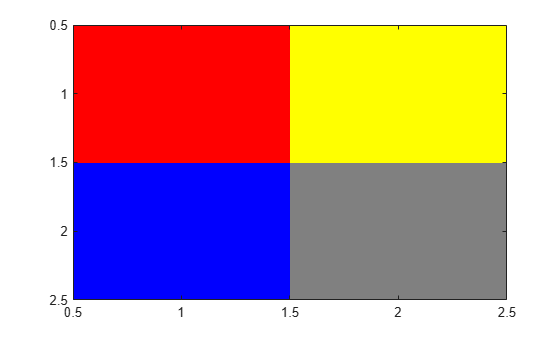# rgb2hsv

Convert RGB colors to HSV

## Syntax

``HSV = rgb2hsv(RGB)``
``hsvmap = rgb2hsv(rgbmap)``

## Description

example

````HSV = rgb2hsv(RGB)` converts the red, green, and blue values of an RGB image to hue, saturation, and value (HSV) values of an HSV image.```

example

````hsvmap = rgb2hsv(rgbmap)` converts an RGB colormap to an HSV colormap.```

## Examples

collapse all

Get a down-sampled version of the `prism` colormap.

`rgb = prism(6)`
```rgb = 6×3 1.0000 0 0 1.0000 0.5000 0 1.0000 1.0000 0 0 1.0000 0 0 0 1.0000 0.6667 0 1.0000 ```

Convert the RGB values in the colormap to HSV.

`hsv = rgb2hsv(rgb)`
```hsv = 6×3 0 1.0000 1.0000 0.0833 1.0000 1.0000 0.1667 1.0000 1.0000 0.3333 1.0000 1.0000 0.6667 1.0000 1.0000 0.7778 1.0000 1.0000 ```

Create a 2-by-2 truecolor image.

```rgb(:,:,1) = [1 1; 0 .5]; rgb(:,:,2) = [0 1; 0 .5]; rgb(:,:,3) = [0 0; 1 .5]; image(rgb);```Convert the image to an HSV array.

`hsv = rgb2hsv(rgb)`
```hsv = hsv(:,:,1) = 0 0.1667 0.6667 0 hsv(:,:,2) = 1 1 1 0 hsv(:,:,3) = 1.0000 1.0000 1.0000 0.5000 ```

## Input Arguments

collapse all

RGB image to convert, specified as an m-by-n-by-3 numeric array. The third dimension of `RGB` defines the red, green, and blue intensity of each pixel, respectively.

Data Types: `single` | `double` | `uint8` | `uint16`

RGB colormap, specified as a c-by-3 numeric matrix with values in the range [0, 1]. Each row of `rgbmap` is a three-element RGB triplet that specifies the red, green, and blue components of a single color of the colormap.

Data Types: `double`

## Output Arguments

collapse all

HSV image, returned as an m-by-n-by-3 numeric array with values in the range [0, 1]. The third dimension of `HSV` defines the hue, saturation, and value for each pixel, respectively, as described in the table.

AttributeDescription
HueValue from `0` to `1` that corresponds to the color’s position on a color wheel. As hue increases from `0` to `1`, the color transitions from red to orange, yellow, green, cyan, blue, magenta, and finally back to red.
SaturationAmount of hue or departure from neutral. `0` indicates a neutral shade, whereas `1` indicates maximum saturation.
Value

Maximum value among the red, green, and blue components of a specific color.

The data type of `HSV` depends on the type of `RGB`:

• If `RGB` is of type `single`, then `HSV` is of type `single`.

• Otherwise, `HSV` is of type `double`.

Data Types: `double` | `single`

HSV colormap, returned as a c-by-3 numeric matrix with values in the range [0, 1]. Each row of `hsvmap` is a three-element HSV triplet that specifies the hue, saturation, and value components of a single color of the colormap.

The data type depends on the type of `rgbmap`:

• If `rgbmap` is of type `single`, then `hsvmap` is of type `single`.

• Otherwise, `hsvmap` is of type `double`.

Data Types: `single` | `double`

 Smith, A. R. “Color Gamut Transform Pairs”. SIGGRAPH 78 Conference Proceedings. 1978, pp. 12–19.